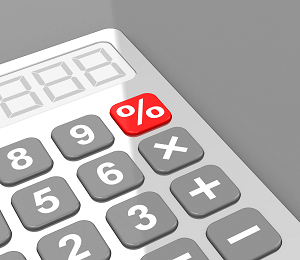Everyday maths 1 (Wales)

Start this free course now. Just create an account and sign in. Enrol and complete the course for a free statement of participation or digital badge if available.

Free course

# 5.2 Finding percentages using a calculator

There are different ways to work out percentages on a calculator. You can work out any percentage on a calculator by dividing by 100 first (to find 1%) and then multiplying the amount by the percentage you need.

If you were asked to work out 20% of 80, you could do the following:

80 ÷ 100 = 0.8

0.8 × 20 = 16

However, most calculators (including those on a mobile phone) will often have a percentage button. The percentage button looks like this:Figure 30 The percentage button on a calculator

To successfully use it when calculating percentages you would enter the sum into your calculator as follows.

If you were asked to find 20% of 80, on your calculator you would input:

80 × 20%

This would give you the following answer:

80 × 20% = 16

If you were asked to find 20% of 80, on your calculator you would input:

20% × 80

This would give you the following answer:

20% × 80 = 16

Different calculators may work in different ways so you need to familiarise yourself with how to use the % button on your calculator.

## Summary

In this section you have learned how to solve problems using percentages, and how to calculate percentage increases and decreases.

FSM_1_CYMRU#### Live in Wales? Free your ambition with a paid part-time course with the OU in Wales.

With grants of up to £4,500* to help with living costs, and tuition fee loans to cover course fees,
now's the time to take that next step.

#### Find out more

*Eligibility rules apply for financial support.